# Longest Line Within a Polygon

13468
10
04-16-2014 01:45 PMNew Contributor III
Hi,
I'd like to model the longest possible line within a (irregular ou regular) polygon feature.
The line should be a 100% inside polygon!!!
Someone knows a tool for that task?
I am using ArcGIS for Desktop 10.2.1.
Tks.
Rafael
Tags (3)
10 RepliesbyMVP Regular Contributor
It sounds like you are wanting to select the longest line feature which is completely within a specific polygon.  Are you looking for a model, a python script, or the instructions for how to do this manually within ArcMap?  In general, I think you would need several tools to address this requirement.  Here are the high-level steps:

** Assumes you have a line feature class and a polygon feature class **

1. Select a single polygon feature, either manually or based on some attribute criteria.

2. Select by Location: select lines from the line feature class which are completely within the polygon feature class (the previously-selected polygon from the step above will honor the selection set of 1 feature)

3. Find the longest feature - this can be handled multiple ways:

• Select by Attribute: select lines from the currently-selected features of the line feature class using MAX(SHAPE.LENGTH) or MAX(SHAPE_LENGTH) depending on your type of data source

• Open the attribute table of the line feature class, choose the option at the bottom to view only the selected features, and sort the SHAPE.LENGTH field (or SHAPE_LENGTH field) in descending order

• Create a new field of DOUBLE (38,8) type and use the Calculate Geometry tool (right click the field from the attribute table) or the Calculate Field geoprocessing tool to calculate the true length of the line features based on the units from the coordinate system of the feature class.

There are likely more ways to handle step 3 than the bulleted options I specified above.  Let me know more about your requirements and I can be more specific about the approach.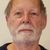Regular Contributor II
Just generate all the lines by connecting vertex pairs of the polygon and find the longest.
Try to avoid iterating around each point combination, think of a process that handles all lines at once.

You can leave out the first and last pair of each point iteration because they will be sides, and you only have to do half because the direction does not matter. You can use python collection permutations to get a unique list of pairs. Then turn them into a list of polylines.

Because a polygon may not be regular, say a banana shape, then you will need a filter to exclude potential lines crossing the boundary for my simple first stab, but there is a test [ a_poly.overlaps(a_line) ] for that in shape objects. Even better you could test them all in one step, using SelectLayerByLocation(..."WITHIN_CLEMENTINI"...) to select remaining candidates to find the max(length). All the tools will take a geometry list instead of a featureclass so it is fast, in memory and efficient enough to iterate over a large number of polygons.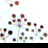Regular Contributor II
I used to do this by building a near table on the vertices and
selecting the pair with the maximum distance; noting that an
isocolese triangle would have two such pairs,
so attention paid to getting the correct pair
(and not the two points across the base)
was worthwhile.

There is a new tool called
MinimumBoundingGeometry In the Management toolbox under Features.

The CONVEX_HULL option adds (among others) the fields
MBG_Length�??The longest distance between any two vertices of the convex hull; these vertices are called antipodal pairs or antipodal points. (It may be found between more than one pair of vertices, but the first found will be used.)
MBG_APodX1�??The x coordinate of the first point of the antipodal pairs.
MBG_APodY1�??The y coordinate of the first point of the antipodal pairs.
MBG_APodX2�??The x coordinate of the second point of the antipodal pairs.
MBG_APodY2�??The y coordinate of the second point of the antipodal pairs.

It does NOT, however, test for interiority...

It does sound like constructing a set of geometry object lines;
testing them WITHIN_CLEMENTINI,
and picking the longest may be what you have to do.Regular Contributor II
I couldn't resist hacking up a script to see what happened.
There were of course a few issues.
The MakeFeatureLayer tool would not take a geometry list, so I resorted to using in_memory featureclasses
The WITHIN_CLEMENTINI query worked fine interactively in ArcMap but chokes on a polygon with more than a dozen vertices in a script. So a new workaround is needed for that. I test each line as it is generated, and then I could also test its length cumulatively so would only have to test for contains if it is a replacement candidate and I only need to keep the longest current line.

This reduced the time per polygon to about 2 seconds. Fairly slow, but it will handle very complex polygons now.

Second Hack:

```#-------------------------------------------------------------------------------
# Name:        LongestLine.py
# Purpose:     find the longest inside line across a polygon
#              Limitations: only single part polygons, no donuts
# Author:      kimo
#
# Created:     29/04/2014
#              30/04/2014 changed crossing test to each features
#               exclude triangles (use trigonometry for this degenerate case)
#               longest line only tested between vertices may need to densify first
#
# Licence:     Creative Commons 3.0 New Zealand
#-------------------------------------------------------------------------------
import arcpy
import sys
import itertools
import datetime
gdb = sys.argv
fcPoly = 'forest'
fcOut = 'fan'
arcpy.env.workspace = gdb
arcpy.env.overwriteOutput = True
begintime = datetime.datetime.now()
desc = arcpy.Describe(fcPoly)
sr = desc.spatialReference
arcpy.env.outputCoordinateSystem = sr
if arcpy.Exists(fcOut):
arcpy.management.Delete(fcOut)
arcpy.management.CreateFeatureclass(gdb,fcOut,"POLYLINE",spatial_reference=sr)
icur = arcpy.da.InsertCursor(fcOut,['ORIGID','SHAPE@'])
with arcpy.da.SearchCursor(fcPoly,["OBJECTID","SHAPE@"]) as cur:
for row in cur:
gPoly = row
id = row
print "Begin id {} parts {} points {} type {}".format(id,gPoly.partCount,gPoly.pointCount,gPoly.type)
if gPoly.partCount == 1 and gPoly.pointCount > 4: # exclude triangles
starttime = datetime.datetime.now()
lstV = []
for part in gPoly:
for v in part:
lstV.append(v)
oCount = 0
pCount = 0
rCount = 0
maxlength = -1
# use itertools to get unique combinations of pairs
# leave in lines coincident with boundary segments
# because they will always be less than max length if > triangle
for vPair in itertools.combinations(lstV[:-1],2): # exclude last duplicated poly vertex
aLine = arcpy.Polyline(arcpy.Array(vPair),sr)
# dont even bother doing spatial test if already shorter for speed
if aLine.length > maxlength :
if gPoly.contains(aLine): # not lines crossing edges
mLine = aLine
maxlength = aLine.length
pCount+=1
else :
oCount+=1
else:
rCount+=1
print "Max swapped {} Overlap rejects {} Already shorter {}".format(pCount,oCount,rCount)
rec = (id,mLine)
icur.insertRow(rec)
msg = "{} {} time {}".format(id,maxlength,datetime.datetime.now() - starttime)
print msg

del icur

print "done",datetime.datetime.now() - begintime

```

I added the original Objectid to be able to relate back to each polygon, geometries do not allow me to keep the original ID so I keep it as a python variable.Regular Contributor II
Since writing my solution I have found an ArcView 3.x solution that shows I have not covered all the cases. You could have a line running through a pair of vertices on a polygon with rentrant sides that extent out each way to the polygon boundary between vertices. I assumed that the longest line would always be coincident with vertices at the ends.

http://www.jennessent.com/arcview/longest_lines.htm

Well done to Jeff Jenness for handling this case in Avenue.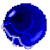by
Occasional Contributor

EDIT: Thanks to @RobertEisler for providing an example showing my transect_type = "PROPER" to be incorrect (the dotted black lines in all the pictures). As far as I am aware, the "BOUNDARY" option is still the optimal algorithm.

As @KimOllivier already noted, only checking vertex-to-vertex is not a sufficient algorithm to solve this problem.

Here is my approach. I am working under the assumption that the longest line will contain two vertices, but does not necessarily end at those two vertices. The user has the option to include the edges of shapes, or require the transect to be entirely internal [this is wrong, see EDIT at the top and replies down below] (red vs. dotted, respectively; sometimes these are the same). Multipart polygons only get one transect generated, so that's why a few shapes appear to be missing lines.

Shapes involving true curves have transects estimated using "densify" to generate actual vertices, so those are only close approximations. See the second screenshot, where the line should presumably be right up against the hole. Would be happy to hear suggestions on this.

These are test polygons, but on a real-world parcel dataset where the number of vertex-to-vertex combinations within a polygon can get into the hundreds of thousands, my version can take a while. Seemed like the bottleneck was converting every vertex coordinate combination to a Polyline object, so I would be very interested in optimizations if anyone has suggestions.

To summarize--script generates lines from all vertex-to-vertex pairs, extends those lines a bit (to cover cases like the castle shape at the top of the first below), clips them back to the polygon boundary, and then finds the longest in the list of lines within the given geometry.

EDIT: The "stopwatch as sw" module in this code is a custom time/message reporting module. Delete it or replace those with standard "print()" if anyone ends up using this.Transect line outputs.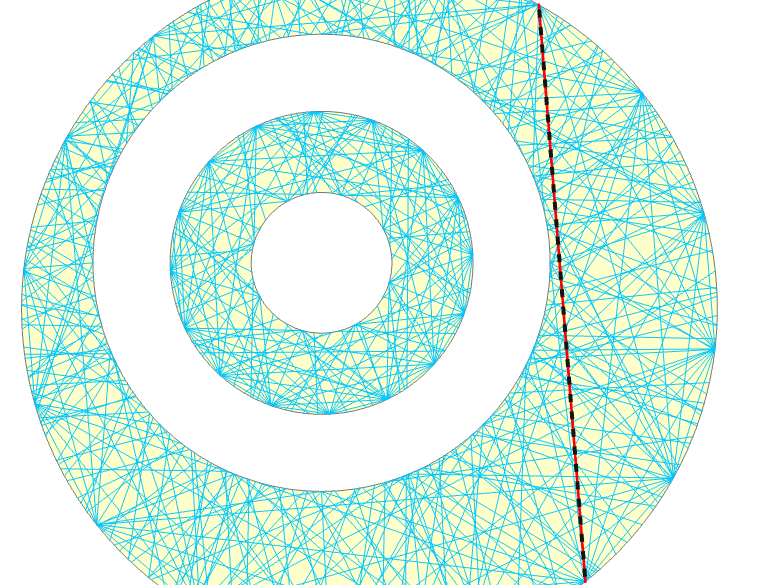Longest transect through a feature composed of curved boundaries.

``````"""LONGEST TRANSECT"""
#---------------------------------------------------------------------------------------#
# IMPORTS
import collections
import itertools
import math
import os
import sys
import traceback
import uuid

import arcpy

import stopwatch as sw
#---------------------------------------------------------------------------------------#
# SETTINGS
arcpy.env.overwriteOutput = True
#---------------------------------------------------------------------------------------#
# INPUTS
polygons = "POLYGON"
polygon_id_fld = "POLY_GUID"
transects = "TRANSECT_BOUNDARY_TEST"

# Boundary = transect can be entire edge. Proper = transect must be witihin polygon.
transect_type = "BOUNDARY"  # ["BOUNDARY", "PROPER"]

# Only save the longest line for each polygon?
#---------------------------------------------------------------------------------------#
# FUNCTIONS
def create_geom_dict(poly_fc, poly_id_fld, generalize=False):
"""Read poly geoms to information dict.
returns {GUID: {POLYGON: <poly_obj>, POINTS: [<pnt_obj>, <pnt_obj>]}, ...}"""
poly_geom_dict = collections.defaultdict(dict)

with arcpy.da.SearchCursor(poly_fc, [poly_id_fld, "SHAPE@"]) as scurs:
# For each row in the FC table (could be single, multi, have holes, etc.).
for guid, geom in scurs:
poly_geom_dict[guid]["POLYGON"] = geom

# Curves to segments; generalize segments to speed process (?).
if geom.hasCurves:
geom = geom.generalize(1)
elif generalize:
geom = geom.generalize(0.5)

# For each part of the geometry:
for i, part in enumerate(geom, start=1):
print(f"  Part {i}")
# For each point in this part of the geometry:
for j, point in enumerate(part, start=1):
if point:
poly_geom_dict[guid].setdefault("POINTS", []).append(point)
else:
print(f"    Point {j:>2}--Null point. Start interior ring.")

return poly_geom_dict

def line_within_polygon(line, polygon, relation):
"""If the line is outside the polygon, or of zero length, return False."""
if not line.within(polygon, relation=relation) or line.length == 0:
return False
else:
return True
#---------------------------------------------------------------------------------------#
# MAIN
stopwatch = sw.Stopwatch()
stopwatch.starttime(msg="PROCESS STARTING")

spat_ref = arcpy.da.Describe(polygons)["spatialReference"].factoryCode

poly_geom_dict = create_geom_dict(poly_fc=polygons, poly_id_fld=polygon_id_fld)

# Remove duplicate points; added due to closing geoms, maybe rings?
for guid in poly_geom_dict:
unique_coors = set((p.X, p.Y) for p in poly_geom_dict[guid]["POINTS"])
poly_geom_dict[guid]["POINTS"] = [arcpy.Point(*coor) for coor in unique_coors]

# Create transect FC. Add Fields.
trans_fc = arcpy.management.CreateFeatureclass(out_path=arcpy.env.workspace,
out_name=transects,
geometry_type="POLYLINE",
spatial_reference=spat_ref)
flds = [("POLY_GUID", "GUID"), ("LINE_GUID", "GUID"), ("LONGEST", "TEXT")]
for fld_name, fld_type in flds:
field_type=fld_type, field_length=1)

stopwatch.stamptime(msg="CREATED TRANSECT FC\n")

# Walk through the dictionary making lines:
# These lists house intermediate geometries; review during testing.
test_multiparts = []
test_extensions = []
for guid, geom_dict in poly_geom_dict.items():
stopwatch.stamptime(msg=f"PROCESSING STARTED ON {guid}")

vert_pairs = len(list(itertools.combinations(iterable=geom_dict["POINTS"], r=2)))
print(f"VERTEX PAIRS TO PROCESS: {vert_pairs}")

line_geoms = []
for p1, p2 in itertools.combinations(iterable=geom_dict["POINTS"], r=2):
line = arcpy.Polyline(inputs=arcpy.Array([p1, p2]))

# WITHIN POLYGON
poly_geom = geom_dict["POLYGON"]
if not line_within_polygon(line, poly_geom, relation=transect_type):
continue

# EXTEND LINE
new_pnts = arcpy.Array()

# Extension distance is somewhat arbitrary. Extend line 1/4 perimeter
#   of the Extent object. Seems to be enough (?).
extend_distance = poly_geom.extent.polygon.boundary().length / 4

angle = math.atan2(p2.Y - p1.Y, p2.X - p1.X)
p1.Y - extend_distance * math.sin(angle)))
p2.Y + extend_distance * math.sin(angle)))

# CLIP TO POLYGON
extended_line = arcpy.Polyline(new_pnts)
clipped_line = extended_line.intersect(poly_geom, 2)
test_extensions.append(extended_line)

# Get all points on line. >2 possible from edge intersections.
merged_array = arcpy.Array()
for part in clipped_line:
for point in part:

# Sort points along X, then Y. Since the line is straight, we don't
#   need to do linear referencing or anything. Keep first/last only.
# Removes needless vertices and reorders points on line.
sort_arr = arcpy.Array(sorted(merged_array, key=lambda p: (p.X, p.Y)))
full_polyline = arcpy.Polyline(arcpy.Array([sort_arr, sort_arr[-1]]))
test_multiparts.append(full_polyline)

# If line extends over white space between multipart polygons.
# isclose() is used here for float precision issues.
if not math.isclose(full_polyline.length, clipped_line.length):
# Retain parts of extended lines that are still within polygons.
# Append each part as a separate line for accurate GDB lengths.
for edge_part in full_polyline.intersect(poly_geom, 2):
line_geoms.append(arcpy.Polyline(edge_part))
else:
# Otherwise, append the new line as-is.
line_geoms.append(full_polyline)

# Export intermediate geometries for testing review.
for geoms, fc_name in [(test_multiparts, "MULTIS"), (test_extensions, "EXTENSIONS")]:
arcpy.management.CopyFeatures(geoms, fc_name)
stopwatch.stamptime(msg=f"{guid} POLYLINES CREATED")

# Sort by length; option to retain only the max length lines, discard the rest.
line_geoms_by_length = sorted(line_geoms, key=lambda x: x.length, reverse=True)
stopwatch.stamptime(msg=f"{guid} TRANSECTS SORTED BY LENGTH")
line_geoms_by_length = line_geoms_by_length[:1]

# Write lines for this polygon to the feature class. Create UUIDs; mark the longest.
flds = ["SHAPE@", "POLY_GUID", "LINE_GUID", "LONGEST"]
with arcpy.da.InsertCursor(trans_fc, flds) as icurs:
for i, line_geom in enumerate(line_geoms_by_length):
if i == 0:
icurs.insertRow([line_geom, guid, uuid.uuid4(), "X"])
else:
icurs.insertRow([line_geom, guid, uuid.uuid4(), None])

stopwatch.stamptime(msg=f"WROTE {guid} TRANSECTS\n")

# Remove records where the geometry is identical.
# This should only happen when an edge is extended through a polygon, and ends
#   up being perfectly coincident with an internal line.
before_lines = int(arcpy.management.GetCount(transects))
arcpy.management.DeleteIdentical(in_dataset=transects, fields="Shape")
after_lines = int(arcpy.management.GetCount(transects))

stopwatch.stamptime(msg=f"{before_lines - after_lines} IDENTICAL LINE(S) REMOVED")
stopwatch.stamptime(msg="PROCESS COMPLETE")``````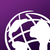New Contributor II

I've put together a model that generates an approximation of the longest line contained within a polygon. It's quite similar to @VinceE 's solution but done through modelbuilder.

It iterates through a feature class and goes through each polygon with a unique ID and then generates points along the boundaries of the polygon at a specified distance, generates a table with all possible combinations of points and the distance between each pair, it then deletes the duplicates that are created as you end up with a row for a to b and one for b to a for each combination of points, from these points it generates lines between each pair, it then clips all the lines to the original polygon and selects the one with the maximum length.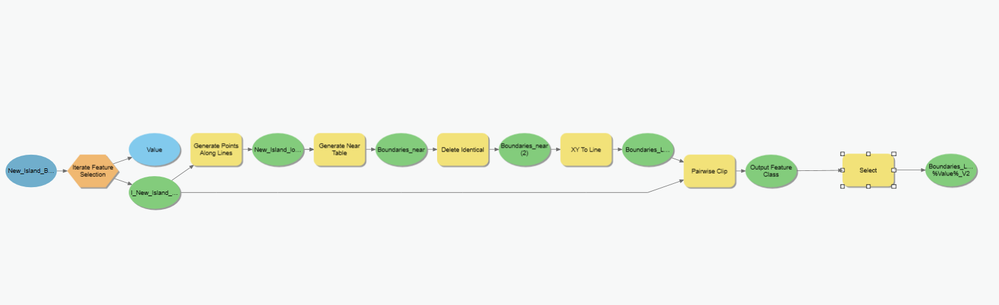Longest line model

See step by step below for how to set up the different parameters in the model.

Iterate Feature Selection (Optional)

Using this means the model will run through the same process for each polygon within a feature class that has a unique ID from the field you have selected. The model can also  be run with just a feature class as input but it will ignore individual polygons within the feature class and will generate a single longest line from the whole feature. E.g I have been using this to find the longest line across each island in a cluster of islands. If using the iterator it will generate a line for each island, if just using feature class which has multiple islands stored in it I will end up with a single line across the longest island.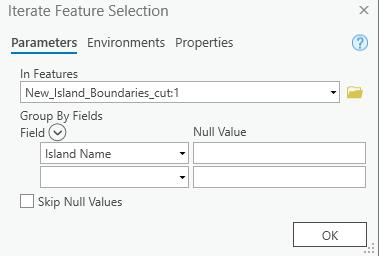Generate points

Input feature is your polygon of interest. Set distance at which points should be placed.Generate near

Input features and Near features are the same layer, the points that were generated in the previous step. Search radius should be large enough to encompass your whole polygon.Delete Identical

Input is the table generated in the previous step.

Deletes half the records as each combination of points is calculated in the near table in one direction and then the other e.g. Y to X is one row and X to Y is a new row which is identical but in the opposite direction.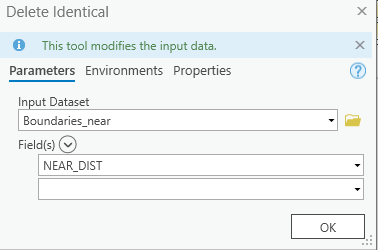XY to line

Input is the table with duplicates removed from the previous step. Make sure to set spatial reference to the same as your map.Pairwise clip

Clips lines generated in previous step to the original polygon. Input features is lines from previous step, Clip features is the polygon you started with.Select

This selects the longest line from the set of lines you have generated and outputs as a feature class. The expression below has the format Field  = (SELECT MAX(Field) FROM (Table)).Tags (1)by
Occasional Contributor